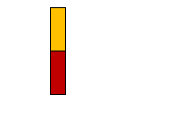# Find the number of different signals that can be generated by arranging at

Question:

Find the number of different signals that can be generated by arranging at least 2 flags in order (one below the other) on a vertical staff, if five different flags are available.

Solution:

Given:5 FlagsWay of generating signal using 2 different flags $={ }^{5} \mathrm{P}_{2}$ (way of selecting 2 things out of 5 things with considering arrangement.)

Way of generating signal using 3 different flags $={ }^{5} \mathrm{P}_{3}$

Way of generating signal using 4 different flags $={ }^{5} \mathrm{P}_{4}$

Way of generating signal using 5 different flags $={ }^{5} P_{5}$

So total number of ways $={ }^{5} P_{2}+{ }^{5} P_{3}+{ }^{5} P_{4}+{ }^{5} P_{5}$

$=20+60+120+120$

$=320$